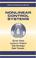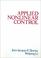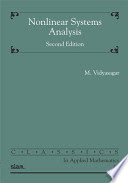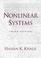### Nonlinear Control Systems

#### Course Description

Dynamic effects due to nonlinearities in systems. Common nonlinear elements in control systems. Phase-plane analysis. Linearization of nonlinear systems. Harmonic linearization. Describing function. Self-oscillations (limit-cycles). Forced oscillations. Harmonic lubrication by dither signal. Stability of nonlinear systems. Feedback linearization. Sliding mode control. Unconventional control methods.

#### General Competencies

Student will learn effects of nonlinearities in control systems. They will be acquinted with methods for analysis of nonlinear control systems as well as how to diminish negative effects of nonlinearities.

#### Learning Outcomes

1. recognize common nonlinear control problems
2. apply some powerfull analysis methods
3. apply some practical design methods
4. discover how nonlinearities can improve system dynamics
5. apply stability analysis methods to real systems
6. identify and remove negative effects that appear in nonlinear control systems

#### Forms of Teaching

Lectures

Mixed type (blackboard+ppt presentations)

Exams

One midterm and one final exam in written form, or an exam in a written and oral form.

Exercises

Examples are given during lectures.

Consultations

Planned with students.

Continuous Assessment Exam
Homeworks 0 % 8 % 0 % 8 %
Mid Term Exam: Written 0 % 16 % 0 %
Final Exam: Written 0 % 16 %
Final Exam: Oral 60 %
Exam: Written 0 % 40 %
Exam: Oral 60 %

#### Week by Week Schedule

1. Introduction in nonlinear systems theory; Basic mathematical and structural models of nonlinear systems; Basic specific and fundamental properties of nonlinear systems
2. The basic properties of nonlinear functions; Typical nonlinear elements; Atypical nonlinear systems
3. Issues of stability; Equilibrium states in the phase plane; The concept of stability of a nonlinear system; Stability of nonlinear systems based on linearized models;
4. Lyapunov stability criterion; Absolute stability of nonlinear systems
5. Geometrical interpretation of the V. M. Popov stability criterion; Stability of forced and unforced systems
6. Linearization methods: Analytical linearization, graphic linearization, harmonic linearization; Describing function; Statistical linearization; Dual describing function
7. Dynamic analysis of nonlinear systems using phase trajectories; Phase trajectories of nonoscillatory processes and periodic solution
8. Midterm exam
9. Dynamic analysis of nonlinear systems using the describing function; Determination of symmetrical and asymmetrical self-oscillations; Stability of periodic solutions
10. Application of the describing function in determination of symmetrical and asymmetrical forced oscillations; Determination of the resonant jump;
11. Control processes in nonlinear systems exhibiting periodical oscillations; Vibrational linearization
12. Determination of transients near a periodic solution; Transient quality diagrams
13. Lie algebra; Feedback linearization - input/output linearization and input/state linearization; Examples
14. Sliding-mode control; Examples
15. Final exam.

#### Study Programmes

Control Engineering and Automation (profile)
Theoretical Course (2. semester)

#### LiteratureZ. Vukić, Lj. Kuljača, D. Đongalić, S. Tešnjak (2004.), Nonlinear Control Systems, New York: Marcel DekkerJ. J. Slotine, W. Li (1991.), Applied Nonlinear Control, New York: Prentice HallM. Vidyasagar (2002.), Nonlinear Systems Analysis, SIAMKhalil, H. K. (2002.), Nonlinear Systems (3rd ed.), New York: Prentice Hall

#### General

ID 34366
Summer semester
5 ECTS
L2 English Level
L1 e-Learning